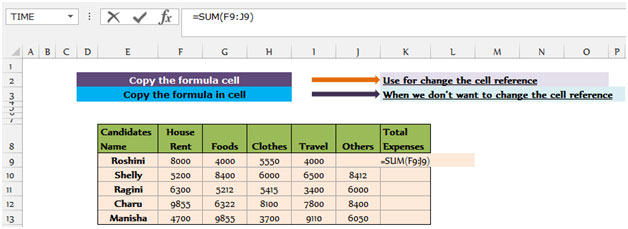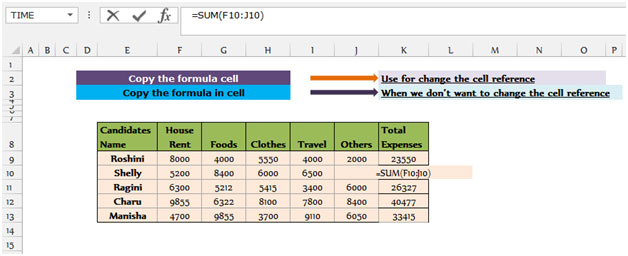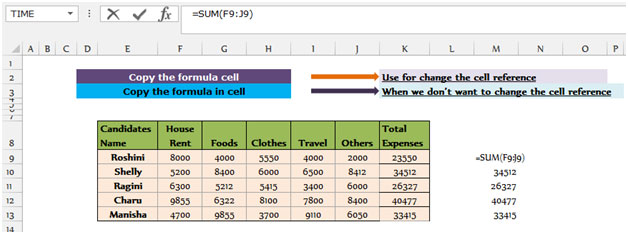# How to copy formulas in Excel

In this article we will learn how to  copy formula from one range or cell to another range or cell in Microsoft Excel.

In simple language, when we copy any formula from one cell to another cell, the cell reference gets changed in Microsoft Excel. However, when we move any formula from one cell to another cell,the cell reference will remain the same.

We can copy  formulas in 2 ways and can get the different results.

Let’s take an example & understand how we can copy function in 2 different ways.

We have a table from Range “E8 to K13” containing monthly expenses details of the candidates. The first row of the table (E8 to K8) contains header, first column “E” is contained candidates’ name and the column “K” is for Total expenses. We have the sum formula for return the total expense to first candidate in cell K9.Change the Cell reference

To get the total expense for the rest of the candidates follow the below given steps:-

• Copy the cell K9 by pressing “CTRL+C” key and paste it into the range K10 to K13 by using the key “CTRL+V” on your keyboard.

Cell K9 which is basically containing sum values for the row number 9, and when we copied the formula and pasted into the below cells, the reference of the range got changed automatically which we do not need to change manually.Copy exact formula:-

After getting the same for all the candidates in range “K9 to K13” using, copying formula, now we do not want the sum to remain in the range “K9 to K13” and wants to change its position to “M9 to M13”. If we copy the range “K9 to K13” by pressing the key CTRL+C and paste it in “M9 to M13” range by pressing CTRL+V, we will see the reference of the range “F9 to J9” got changed to “H9 to L9” which we do not want.

To get the actual range, which is “F9 to J9” to remain same when we make changes in the position of Total Expenses column.

• We have to first select the formula cell.
• Press the key F2, copy the formula by using the key Ctrl+C and paste in the cell M9 by using the key Ctrl+V on your keyboard.
• We will see, the references of the sum, are still same as it was in cell K9.
• Copy the cell M9 by pressing “CTRL+C” key and paste it into the range K10 to K13 by using the key “CTRL+V” on your keyboard.So in simple words, if we want to change the cell reference automatically, we should use copying function, and when we do not want the reference get changed, we should use moving function.